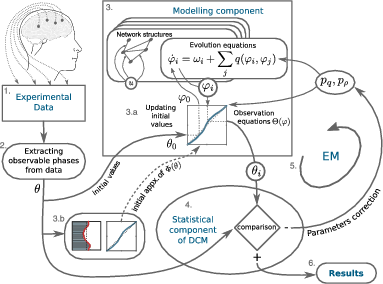# Reconstruction of effective connectivity in the case of asymmetric phase distributions

The interaction of different brain regions is supported by transient synchronization between neural oscillations at different frequencies. Different measures based on synchronization theory are used to assess the strength of the interactions from experimental data. One method of estimating the effective connectivity between brain regions, within the framework of the theory of weakly coupled phase oscillators, was implemented in Dynamic Causal Modelling (DCM) for phase coupling (Penny et al., 2009). However, the results of such an approach strongly depend on the observables used to reconstruct the equations (Kralemann et al., 2008). In particular, an asymmetric distribution of the observables could result in a false estimation of the effective connectivity between the network nodes.
In this work we built a new modelling part into DCM for phase coupling, and extended it with a distortion function that accommodates departures from purely sinusoidal oscillations.
By analysing numerically generated data sets with an asymmetric phase distribution, we demonstrated that the extended DCM for phase coupling with the additional modelling component, correctly estimates the coupling functions.
The new method allows for different intrinsic frequencies among coupled neuronal populations and provides results that do not depend on the distribution of the observables.
The proposed method can be used to analyse effective connectivity between brain regions within and between different frequency bands, to characterize m:n phase coupling, and to unravel underlying mechanisms of the transient synchronization.Figure. The scheme of the extended DCM for phase coupling. In DCM, raw data (1) or some pre-processed observables (2) obtained from an experiment are “compared” with the observables generated by the modelling component of DCM (3) in the statistical component of DCM (4) using the Bayesian model inversion. The interactive update of the parameter values using the EM algorithm is displayed by the feedbackloop arrow (5). This interation continues until the values of the parameters of the model best fit the data (6). A non-linear observable equation, update of the initial values (3a), and initial approximation of the non-linear observable function (3b) are the new extensions into DCM for phase coupling.

Publication:

Yeldesbay, A., Fink, G.R., & Daun, S. (2019). Reconstruction of effective connectivity in the case of asymmetric phase distribution. Journal of Neuroscience Methods, 317, 94-107.